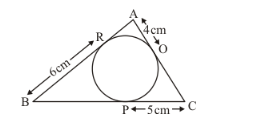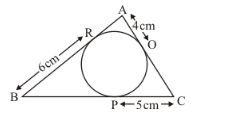# In the given figure, the perimeter of Δ ABC is

Question:

In the given figure, the perimeter of Δ ABC is(a) 30 cm

(b) 60 cm

(c) 45 cm

(d) 15 cm

Solution:

We know that tangents from an external point will be equal in length.Therefore,

AQ = AR

AQ is given as 4 cm. Therefore,

AR = 4

Similarly,

PC = CQ

PC = 5

Therefore,

CQ = 5

Similarly,

BP = BR

BR = 6

Therefore,

BP = 6

Now we can find out the perimeter of the triangle.

Perimeter = AB + BC + CA

From the figure we have,

Perimeter = AR + RB + BP + PC + CQ + QA

Perimeter = 4 + 6 + 6 + 5 + 5 + 4

Perimeter = 30 cm

Therefore, option (a) is the correct answer.